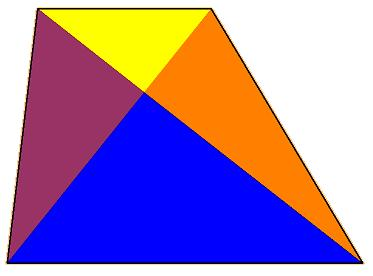#### You may also like### At a Glance

The area of a regular pentagon looks about twice as a big as the pentangle star drawn within it. Is it?### Six Discs

Six circular discs are packed in different-shaped boxes so that the discs touch their neighbours and the sides of the box. Can you put the boxes in order according to the areas of their bases?### Equilateral Areas

ABC and DEF are equilateral triangles of side 3 and 4 respectively. Construct an equilateral triangle whose area is the sum of the area of ABC and DEF.

# Trapezium Four

##### Age 14 to 16Challenge Level

You may wish to use GeoGebra to explore this problem.

What makes a shape a trapezium?

The diagonals of a trapezium divide it into four parts.• Can you create a trapezium where two of those parts are equal in area?

• Can you create a trapezium where three of those parts are equal in area?

• Can you create a trapezium where all four parts are equal in area?

In each case can you explain how much freedom you have?

Or, if any of those challenges are impossible, say why you think that.